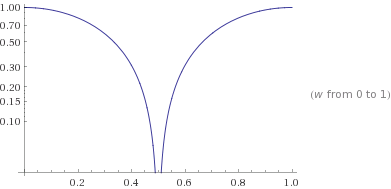## Sunday, January 31, 2016

### The Boxcar Digital Lowpass Filter

In embedded systems it is quite common to need a little bit of filtering on analog quantities. Programmers sometimes feel they need the filtering to help smooth any analog conversion noise. Two type of filters commonly used are the Boxcar and the Exponential filter. Both are simple to implement. Its important to understand the impact these filters have on delay and frequency response, and to evaluate their comparative effectiveness.

The Boxcar filter is a simple averaging filter. Technically it is an FIR filter. Typically you would obtain your next analog value, add it to the last n-1 values read, then divide by n. It's just a simple average of the last few values read.

A common value for n is 4. The Z transform of a filter with n=4 is H(z) = ¼ (z3+z2+z+1)/(z3) . There are three poles at the origin in the Z domain. Thus this filter is stable. There is one real zero at -1, and two complex conjugate zero's at +i and -i. The (normalized) frequency response is given by H(e) = magnitude(¼ + ¼e-jω+ ¼e-j2ω+¼e-j3ω).
Click on the link to use Wolfram-Alpha to plot this function. In the following image (copied from Wolfram) we see several cycles of normalized frequency response including mirroring above half the sample rate.   The mirroring is how aliasing shows up in the frequency response.Box Car Filter Normalized Response.  N=4.

Note that the horizontal axis is in Hz. The sampling frequency is 1Hz.  (The response is normalized to 1 Hz.)  One half the sample rate is 0.5Hz - the Nyquist rate. What you are seeing above 0.5Hz is a periodic mirror of the response. But lets focus on the response below 0.5 Hz.  How UGLY for a low pass response.  Lets try to plot the response for n=2. With this filter all we do is add the current and previous samples and divide by 2. The characteristic equation is Y = ½(X + Xz-1) .  The transfer function is H(z) = ½(1+z-1) = ½(1+z)/z . This has a single pole at 0 and a zero at -1. Thus its stable. The normalized frequency domain function H(e) = ½(1+e-jω).  Its normalized for a sample time of 1sec. Click here to have Wolfram Alpha plot the response.Box Car Filter Normalized Response.  N=2.
This looks more like a low pass response. Note that the horizontal axis this time is in Hz. Since the sample time is a normalized 1second, then the sample frequency is 1.0 Hz, and the Nyquist frequency is 0.5 Hz.

In my next Laboratory Note we will look at the Exponential Filter. This is actually my favorite and I will show you why!

1.This comment has been removed by a blog administrator.

2.This comment has been removed by a blog administrator.

3.This comment has been removed by a blog administrator.

4.This comment has been removed by a blog administrator.